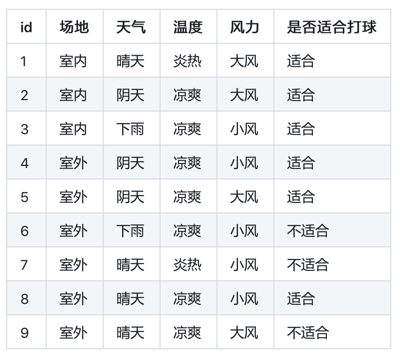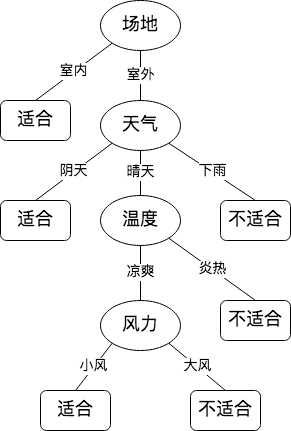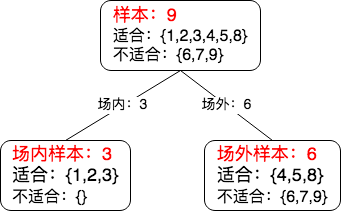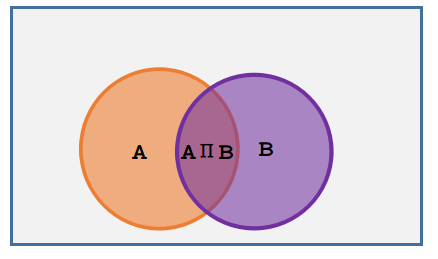### 决策树回顾• 信息增益：在 ID3 决策树中使用
• 信息增益率：在 C4.5 决策树中使用
• 基尼系数：在 CART 决策树中使用• 划分前: 样本 9
• 适合：{1,2,3,4,5,8}，占比 6/9
• 不适合：{6,7,9} 占比 3/9
• 按类别划分后：
• 室内样本 3
• 室内适合：{1,2,3} 占比 3/3
• 室内不适合：{} 占比 0/3
• 室外样本 9
• 室外适合：{4,5,8} 占比 3/6
• 室外不适合：{6,7,9} 占比3/6

### 一、信息增益

ID3 决策树采用信息增益选取最优划分属性。

##### 1. 回顾信息熵

E n t ( X ) = − ∑ x ∈ X p ( x )   l o g   p ( x ) Ent(X) = - \sum_{x \in X} p(x)\,log\,p(x)

##### 2. 从熵说到信息增益

1. 划分之前计算事件的熵：Ent(X)
2. 按照属性 A 划分后再次计算事件的熵：Ent(X|A)
3. 则 Ent(X) - Ent(X|A) 就是划分之后熵被消除了多少。

##### 3. 求解条件熵 Ent(X|A)

E n t ( X ∣ A ) = ∑ a ∈ A p ( a )   E n t ( X ∣ A = a ) Ent(X|A) = \sum_{a \in A} p(a)\,Ent(X|A=a)

# 场地" 划分举例：

Ent(X|场地) =
p(室内) * Ent(X|场地=室内)
+
p(室外) * Ent(X|场地=室外)


E n t ( X ∣ A = a ) = − ∑ x ∈ X p ( x ∣ A = a )   l o g   p ( x ∣ A = a ) Ent(X|A=a) = - \sum_{x \in X} p(x|A=a)\,log\,p(x|A=a)

##### 4. 条件概率

p ( a ∣ b ) = p ( a b ) p ( b ) p(a|b) = \frac{p(ab)}{p(b)}b 条件下 a 的概率就是 ab 交集的概率除以 b 的概率，简单理解就是从 b 中找 a, 所以 p(a|b) 的竖线理解成除号就可以了。

场地" 划分举例：

p(适合|室内)
= p(室内适合) / p(室内) = (3/9) / (3/9)
= 3/3 = 1

p(不适合|室内)
= p(室内不适合) / p(室内) = (0/9) / (3/9)
= 0/3 = 0

p(适合|室外)
= p(室外适合) / p(室外) = (3/9) / (6/9)
= 3/6 = 1/2

p(不适合|室外)
= p(室外不适合) / p(室外) = (3/9) / (6/9)
= 3/6 = 1/2


##### 5. 利用信息增益选择分支

G a i n ( X , A ) = E n t ( X ) − E n t ( X ∣ A ) Gain(X,A) = Ent(X) - Ent(X|A)

E n t ( X ) = − ∑ x ∈ X p ( x )   l o g   p ( x ) Ent(X) = - \sum_{x \in X} p(x)\,log\,p(x)

E n t ( X ∣ A ) = ∑ a ∈ A p ( a )   ∗   E n t ( X ∣ A = a ) = ∑ a ∈ A p ( a )   ∗   [ − ∑ x ∈ X p ( x ∣ A = a )   l o g   p ( x ∣ A = a ) ] \begin{aligned} Ent(X|A) &= \sum_{a \in A} p(a)\,*\,Ent(X|A=a) \\ &= \sum_{a \in A} p(a)\,*\,[- \sum_{x \in X} p(x|A=a)\,log\,p(x|A=a)] \end{aligned}

# "场地" 划分举例：

Gain(X,场地) = Ent(X) - Ent(X|场地)

1. 计算Ent(X)
Ent(X) = - [p(适合) logP(适合) + p(不适合) logP(不适合)]
= - [6/9 log(6/9) + 3/9 log(3/9)]
= 0.2764

2. 计算Ent(X|场地)
Ent(X|场地) =
p(室内) * Ent(X|场地=室内)
+
p(室外) * Ent(X|场地=室外)

(1). p(室内) = 3/9

(2). p(室外) = 6/9

(3). Ent(X|场地=室内)
= - [p(适合|室内) logP(适合|室内) + p(不适合|室内) logP(不适合|室内)]
= - [1 log1 + 0 log0]
= 0

(4). Ent(X|场地=室外)
= - [p(适合|室外) logP(适合|室外) + p(不适合|室外) logP(不适合|室外)]
= - [1/2 log(1/2) + 1/2 log(1/2)]
= 0.3010

Ent(X|场地) = 3/9 * 0 + 6/9 * 0.3010
= 0.2007

// 信息增益
Gain(X,场地) = Ent(X) - Ent(X|场地)
= 0.2764 - 0.2007
= 0.0757


### 二、信息增益率

C4.5 决策树采用信息增益率选取最优划分属性。

##### 1. 为什么要用信息增益率

• 如果各个属性的值的个数比较类似，缺陷并不明显
• 比如：场地{室内、室外}，风力{大风、小风}，属性值都只有两个
• 如果各个属性的值的个数差异较大，会发现信息增益偏向值个数多的属性
• 比如：场地{室内、室外}，风力{台风、狂风、大风、小风、微风、无风}，属性值一个是两个一个是六个

##### 2. 什么是信息增益率

G a i n R a t i o ( X , A ) = G a i n ( X , A ) I V ( A ) GainRatio(X,A) = \frac{Gain(X,A)}{IV(A)}

I V ( A ) = − ∑ a ∈ A p ( a ∣ A )   l o g   p ( a ∣ A ) IV(A) = - \sum_{a \in A} p(a|A)\,log\,p(a|A)

• IV: (intrinsic value), 表示属性的固定值, p(a|A) 表示a在A中占得比例。

# 场地" 划分举例：

Gain(X, 场地) = 0.0757

IV(场地) = -[p(室内|场地) log p(室内|场地)
+
p(室外|场地) log p(室外|场地)]
= -[3/9 log (3/9) + 6/9 log (6/9)]
= 0.2764

GainRatio(X, 场地)
= Gain(X, 场地) / IV(场地)
= 0.0757 / 0.2764
= 0.2739

##### 3. 信息增益率完美吗

• 信息增益偏向值个数多的属性
• 信息增益率偏向值个数少的属性

### 三、基尼系数

CART 决策树采用基尼系数选取最优划分属性。

G i n i ( X ) = ∑ x ∈ X p ( x )   ( 1 − p ( x ) ) = 1 − ∑ x ∈ X p ( x ) 2 \begin{aligned} Gini(X) &= \sum_{x \in X} p(x)\,(1-p(x)) \\ &= 1 - \sum_{x \in X} p(x)^2 \end{aligned}

G i n i ( X , A ) = ∑ a ∈ A p ( a )   G i n i ( X ∣ A = a ) = ∑ a ∈ A p ( a )   ( 1 − ∑ x ∈ X p ( x ∣ a ) 2 ) \begin{aligned} Gini(X,A) &= \sum_{a \in A} p(a)\,Gini(X|A=a) \\ &= \sum_{a \in A} p(a)\,(1 - \sum_{x \in X} p(x|a)^2) \end{aligned}

# "场地" 划分举例：

Gini(X,A)
= p(室内) * Gini(X|场地=室内)
+
p(室外) * Gini(X|场地=室外)
= p(室内) * {1 - [p(适合|室内)^2 + p(不适合|室内)^2]}
+
p(室外) * {1 - [p(适合|室外)^2 + p(不适合|室外)^2]}
= 3/9 * {1 - [1 + 0]}
+
6/9 * {1 - [1/4 + 1/4]}
= 0.3333


### 后记